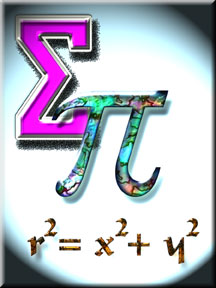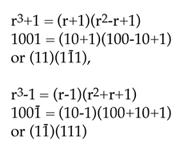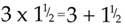Pebbles on the Beach...
 by way of introduction to the Numbers pages ... I used to cycle back after work; which could be quite a chore, especially if the weather was bad. There was a long haul up the road and over the motorway bridge, and to take my mind off it I settled into the habit of thinking out a problem, or carrying out some mechanical routine calculation to occupy my mind. Sometimes I would pick a passing car at random and factorise its licence number, to check if this was a prime. I used different methods, depending on the number and any patterns I might recognise, and developed some simple rules - for example, for division by 7 or 13 - but much of the time I relied on using easily remembered multiples and subtracting them from the number, or the number from the multiple. Suppose the number was 971. Firstly, I wouldn't need to test beyond 31 (312 = 961); I would first check for the prime 3 ( using the sum of digits rule) , then 11 (difference of digits), since these two are easy. Then 7 - take 700 off 971 leaves 271, and 7 x 40 = 280, 280 - 271 = 9 so 7 won't go. Try 13...... 7 x 13 = 91, subtract 910 from 971 leaves 61, and since 5 x 13 = 65, 13 won't go. Try 17...... 3 x 17 = 51, subtracting 51 from 971 leaves 920, and 92 being 2 x 2 x 23 means 17 won't go. This shows the sequence of thoughts that would go through my mind while pedalling. At times I might use a recipe - such as that for dividing by 7 - which I later realised as being derived from a residue system. The method used depended on the number I was testing. The reason for looking for recipes was because Jean Essig stated in "Douze Notre Dix Futur" that there was "no easy rule" for testing divisibility by 7 in base twelve. You'll find some of these "recipes" among the html and PDF documents on this site. Sometimes I would factorise a number assuming it was written in base twelve. Finding patterns in multiples makes this easier; for example 5 x *25 (* for a base twelve number) is *101. Given a three-figure license number subtracting multiples of *101 will reduce it to a number of two digits or less, and these are easy to check. From this factorising I developed a method of checking divisibility by using power residues. There are other methods for checking divisibility, by G.S.Terry and I.G.Valevski. I don't claim I found any new method; I have since found some of them in text books. (L: articles on factorising, and tests of divisibility) Now, though, with the post 2000 system of numbering license plates with year numbers and assorted letters there are fewer vehicles on the road with the old plates and interesting numbers ... At other times, tiring of factors, I would choose a different problem - such as constructing an algorithm to program the Knight's Tour, or reason out some problem that had occurred during the day, or develop an idea such as (3+4)3 = 343, derived from noticing a particular feature of some car number. There are some more numbers like 343 on "ThreeFourThree" I find the patterns in numbers fascinating. Playing with different bases is part of this, and sometimes a number approach, in preference to an algebraic one, produces more interesting results. For example, ignoring the prime 2, "is x2+1 ever prime" could be put in numbers as "in what bases is '101' a prime? Algebraically, if x is odd, so is x2, so x2+1 is even; in numbers Q(101) = 2, so 101 in an odd base is even; both statements imply that x has to be even for x2+1 to be add, but show the two aspects of the same problem, each appealing in its own way. [The function Q(x) represents the sum of the digits used in the number x; so, for example, Q(102) =1+0+2 =3.] (L: repunit numbers) Similar ideas occur with "repunit" (repeated unit) numbers, linking different parts of mathematics. In numbers "11", "111", "1111" etc; in algebra r+1, r2+r+l, r3+r2+r+1 etc. In an odd-number base a repunit number N cannot be prime if Q(N) is even - which eliminates 11, 1111 etc. Also a repunit number looks very much like the pattern after the 0 in 0.11111... or 1/(r-1) in base r - which links with algebraic expansions of 1/(r-1), geometric progressions and on via Fermat and into Number Theory.(L) Alternatively, you can go from the algebra to the number: x2+1 has no real factors, but "101" can have factors (101 base eight = 3 x 3) What about 1001, 10,001 etc? These have factors because algebra tells us r3+l, r4+1 have factors - (r+1)(r2-r+1) for r3+1 for example. If we allow negative digits with positional notation (a system known as "Reverse Notation" or "Two-Way Notation" we can show these expansions in numbers as:as an introduction to Two-Way notation, you might like the PDF file Backwards & Forwards (87K) There is a wealth of fascination in numbers. Even simple, well-known facts, such as the sum of the odd numbers giving the squares - on to triangle and other figurate numbers - or the sum of the cubes producing the squares of the triangle numbers; and on into Pythagoras, Fibonacci, patterns and sequences for pi, e ... a lifetime seems hardly enough to absorb even the basics of it all, even without going outside the realm of simple number. Here are some articles you can read on site: Egyptian Fractions.. some thoughts Palindromic Primes Spots before my eyes Prime Reversals 153 - Why 153 is a special number Base Work A Number Sequence Puzzle Oddments a selection of number themes Squares two little articles on squares Millions and Billions about the two systems of naming large numbers Two for the calculator (might be a bit old-fashioned?) fractionals changing them from base to base Cube and higher roots by using the square root key Here are two (short) PDFs on primes (both also referenced on the dozenal page), one a list of primes in base twelve, and the other an article on prime forms in different bases and in general. And for a little something on number patterns, you might like the PDF file Find the next term..(96K) Here's a puzzle (PDF file) to which I might still have the answers: NB: There's a mistake in the Crossnumber clues; 26a = 40 - 13a should read 26a = 40a - 13a. (My thanks to "danielb" for pointing this out). Some odd fact will catch my attention - and provide a topic for investigation; it could be a remark, a car number, a problem in an old text book, or just, for example, a simple fact likeis quite sufficient to launch an investigation into solutions for ab = a+b. Or here's another oddment, where an idea can be extended, through number bases, and generalised. If we take a 3-digit number, say 732, reverse it, and subtract the reversal, then reverse the answer and add that to the result: 732 - 237 = 495, 495 + 594 =1089. In base ten we always finish with the answer 1089. In base twelve 732 - 237 = 4E7, 4E7 + 7E4 = 10TE - and in base twelve we always get 10TE. (Until we can embed special fonts with new symbols for ten and eleven, T stands for ten and E for eleven). The numbers 1089 and 10TE, are both of the form r3+r2-r-1 (number base r); so we can predict that the same recipe will produce 1056 in base seven, 1023 in base four, and so on. What is not as obvious, but just as true, is that the recipe will produce a constant answer even in a mixed base calculation. (L: article expanding this).(Dudeney has a proof in his "Mathematical Recreations") I would like discover something new (or perhaps rediscover something forgotten by everyone ... ); my pupils used to joke about the day "Fergie's Feorem" would make me famous. I haven't found any theorems that are new; but there might be something in my researches and investigations that might illuminate, for some other investigator, a corner of number theory or number lore which hasn't been noticed yet. Mind you, having discovered that so many problems have been resolved by using a computer, the question now is - what is there still left to prove/discover? Has the computer made it all so easy that there's nothing left for the amateur mathematician to find out??? When younger, and working on reciprocals and their patterns - a fascinating field - I designed the formula:for any base "10", which expresses the reciprocal directly in figures as a power series, e.g. 1/98 = 0·01 02 04 08 16 32 ... (base ten) 1/ET = 0·01 02 04 08 14 28 ... (twelve) 1/65 = 0·01 02 04 11 22 44 ... (seven) which is really just an adaptation of the Binomial Theorem - but that was a theorem I didn"t know at the time. I used to calculate reciprocals in different bases with pencil and paper; then, noticing the patterns, designed the formula, working, as usual, from the numbers and the patterns to the theory. (I have a lot more on these, but the typing and correcting will take a while before I can link it in here). If I present a proof anywhere, the odds are that the patterns came first, and the proof was added afterwards to make the presentation more "correct" mathematically. If the conclusions look right, and the patterns are there, I am usually quite happy to let someone else, more interested in proofs, get on with the formal side of proving the results. I still enjoy working out a square root by the long method with pencil and paper; and "sums" like working out the cost of 3lb 6oz of tea at 4s6d per lb; or just picking a problem at random from one of my (pre-decimal currency) arithmetic books or an algebra book. I have jotted down a few ideas over the years, and what you read here are the bits I've edited and rewritten for this website. There are some more to develop and write, but I shall put them, and revisions of any work here, on the DSGB site in future. All comments and suggestions welcome! If you started on this page (with no panel of square buttons on the left) and would like to see the other topics on this site Home will take you to our Home Page